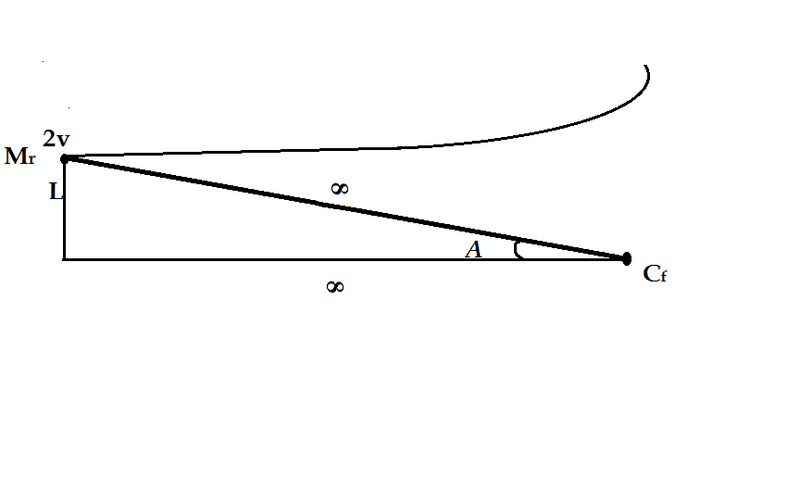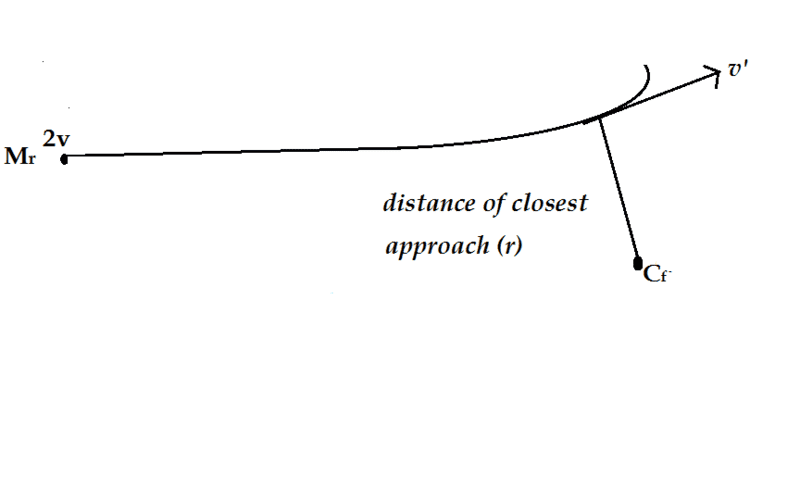# Distance of closest approach of like charge

## Homework Statement

A proton and an α -particle are projected with velocity v=√$\frac{e^{2}}{4∏εmL}$ each from infinity as shown.The perpendicular distance between their initial velocities is L .Let the distance of their closest approach be D .

Given .Find (8D/L - 5)^2

Details and assumptions

1)mass of proton=m,charge=+e

2)mass of α-particle=4m,charge=2e

## The Attempt at a Solution

As the particles are located at infinite distance from each other so I think dist.L will not matter much.
I tried to use reduced mass problem here.
Here reduced mass(M) =4m/5 let it be at infinite distance from origin O.
What should be the initial velocity of reduced mass here? Is it 2v.(I used 2v here)
Lat it be at a distance D from O after some time.
At D all its kinetic energy wiil be converted to potential energy so
Mv^2/2=k2e^2/R
On putting values and after doing some calcilations I got
4D=5L
I got the value of 25.Am I right?
I think I am not.

This is the link of figure.
d3pq38zxuosm5i.cloudfront.net/solvable/2676130fa9.92b5dbd0d6.O7DvUt.png

Last edited:

## Answers and Replies

BiGyElLoWhAt
Gold Member
I'm not following. What do you mean by the perpendicular distance between their velocity? You've got misspellings and random punctuation out the ***.
I would suggest cleaning this post up a bit.

I'm not following. What do you mean by the perpendicular distance between their velocity? You've got misspellings and random punctuation out the ***.
I would suggest cleaning this post up a bit.
A proton and an α -particle are projected with velocity v=√e^2/4∏εmL each from infinity to each other as shown in figure.The perpendicular distance between their initial velocities is L .Let the distance of their closest approach be D .

The direction of velocities of two particles are opposite to each other but the particles do not lie on the same line.The perpendicular distance between their initial velocities means the perpendicular distance between the direction of their initial velocities.
I think it will be clear from the figure here. http://d3pq38zxuosm5i.cloudfront.net/solvable/2676130fa9.92b5dbd0d6.O7DvUt.png
Sorry for the inconvenience.

ehild
Homework Helper

## Homework Statement

A proton and an α -particle are projected with velocity $v=√\frac{e^{2}}{4∏εmL}$
Do you mean $v=\sqrt{\frac{e^{2}}{4\pi εmL}}$ ?

each from infinity as shown.The perpendicular distance between their initial velocities is L .Let the distance of their closest approach be D .

Given .Find (8D/L - 5)^2

Details and assumptions

1)mass of proton=m,charge=+e

2)mass of α-particle=4m,charge=2e

## The Attempt at a Solution

As the particles are located at infinite distance from each other so I think dist. L will not matter much.

It does :tongue: There is angular momentum of the system, and you have to take its conservation into account.

I tried to use reduced mass problem here.
Here reduced mass(M) =4m/5 let it be at infinite distance from origin O.
What should be the initial velocity of reduced mass here? Is it 2v.(I used 2v here)
Lat it be at a distance D from O after some time.
At D all its kinetic energy wiil be converted to potential energy so
Mv^2/2=k2e^2/R

No. the radial component of the velocity is zero, but but not the whole velocity.

ehild

#### Attachments

•closestapp.JPG
3.2 KB · Views: 391
Do you want me to apply law of conservation of momentum.

I did not have knowledge about angular momentum and torque, so I explored about them yesterday.
I found that these quantities are associated with rotational motion of system.
In rotational motion the axis of system does not move and other particles of the system move around it.

Are concepts of rotational motion related to this question? If yes,then please explain how.

You want me to use angular momentum here but angular momentum is calculated w.r.t a fixed point.
Where is this 'fixed point' in this question?

I don't understand why the kinetic energy is not zero at the distance of closest approach of particles.As particles stops momentarily at the distance of closest approach so Kinetic energy should be 0 at that point.
Thank you ehild.

Do you mean $v=\sqrt{\frac{e^{2}}{4\pi εmL}}$ ?
Yes. I didn't get the symbol of 'pi'

ehild
Homework Helper
Do you want me to apply law of conservation of momentum.
No, I want you to use conservation of angular momentum. It is conserved in a central force field.
You can define angular momentum for a particle with respect to a fixed origin.
It is ##\vec L= \vec r \times \vec p ##, the cross product of the position vector with the linear momentum. The time derivative of the angular momentum is equal to the torque. A central force has zero torque, so the angular momentum is conserved in a central force field. Your virtual particle moves in a central force field, where the force acts away from a repulsive centre.

You want me to use angular momentum here but angular momentum is calculated w.r.t a fixed point.
Where is this 'fixed point' in this question?

I don't understand why the kinetic energy is not zero at the distance of closest approach of particles.As particles stops momentarily at the distance of closest approach so Kinetic energy should be 0 at that point.
Thank you ehild.

If you have the real particles you can choose the fixed point at the CM when they are at the closest approach. In the reduced mass approach, you have a fixed centre of force, determine the angular momentum with respect to it.

The kinetic energy becomes zero at closest approach if the particles move along the same line. Here they move along curved lines which do not intersect. See picture. If they stopped somewhere, they would move along a straight line away from the CM and each other and the angular momentum of the system would be zero.

#### Attachments

I have made the figure ehild.Please see that.

I have some problem in finding the initial angular momentum.
L=mvrsinθ.Here r=∞.

But from the figure
cosA=∞/∞=1
so A=0.
So the angle between velocity vector and position vector=180(joining both vector from tail to tail)
but sin180=0
So initial angular momentum is 0.Is it right..
This is the figure.

ehild
Homework Helper
You can not calculate with infinity like that. ∞/∞ is not 1. Think about x/x2, both the numerator and the denominator goes to infinity, what about the ratio?
You never reach infinity. It is only a limit - you can increase something more and more but it is still finite.

Initially the point is very far away but still at a finite distance R. Rsin(A)=L.
So the angular momentum of the virtual particle is μ(2v)L.

ehild

You can not calculate with infinity like that. ∞/∞ is not 1. Think about x/x2, both the numerator and the denominator goes to infinity, what about the ratio?
You never reach infinity. It is only a limit - you can increase something more and more but it is still finite.

Initially the point is very far away but still at a finite distance R. Rsin(A)=L.
So the angular momentum of the virtual particle is μ(2v)L.

ehild
Ok I get that.
For calculating final angular momentum.
I have made a figure.

L=mvrsinθ(θ in this case should be equal to 90 I think)
Lf=μv'r.
Is it right?ehild
Homework Helper
Ok I get that.
For calculating final angular momentum.
I have made a figure.

L=mvrsinθ(θ in this case should be equal to 90 I think)
Lf=μv'r.
Is it right?

View attachment 71708

Yes. The initial angular momentum is μVL, (V=2vo) and the final one is μv'r, r is the distance of closest approach (the problem text uses D for it). The initial angular momentum is equal to that at the closest approach.

ehild

Yes. The initial angular momentum is μVL, (V=2vo) and the final one is μv'r, r is the distance of closest approach (the problem text uses D for it). The initial angular momentum is equal to that at the closest approach.

ehild

μ2vL=μv'D
or 2vL=v'D.
If I use law of conservation of energy and substitute v'=2vL/D.Will I get the answer?

ehild
Homework Helper
μ2vL=μv'D
or 2vL=v'D.
If I use law of conservation of energy and substitute v'=2vL/D.Will I get the answer?

I hope.ehild

v'=$\frac{2vL}{D}$

$\frac{4μv^{2}}{2}$=$\frac{k2e^{2}}{D}$+$\frac{4μv^{2}L^{2}}{2D^{2}}$

On simplifying and putting the values of v,μ=4m/5 and k.
I got

4D$^{2}$=5DL+4L$^{2}$.

I am not able to find (8D/L - 5)^2

ehild
Homework Helper
Divide the whole equation with L2. You get a quadratic equation for D/L. Write the quadratic formula, but do not evaluate. What do you get?

ehild

Divide the whole equation with L2. You get a quadratic equation for D/L. Write the quadratic formula, but do not evaluate. What do you get?
ehild
Is it 89?

ehild
Homework Helper
It looks all right.

ehild

•1 person
It looks all right.

ehild
Thank you ehild.You are the best.

ehild
Homework Helper
Thank you, but it is an overstatement:shy:

ehild